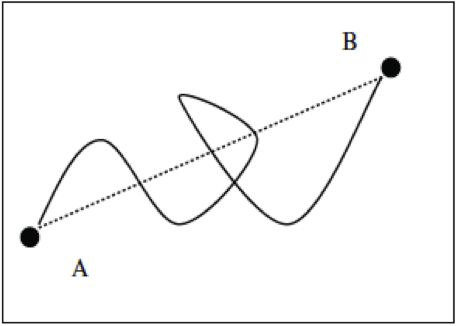The Curviness Tool

How does the curviness tool work?

• The curviness number is calculated by dividing the distance along the path from point A to point B (solid line) by the straight-line distance between point A and point B (dashed line).• The closer this number is to 1, the straighter the animal is travelling. The higher the value is, the curvier the animal’s path is.